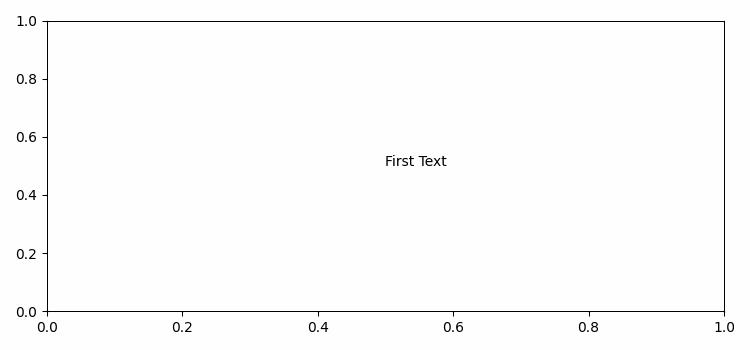# How to refresh text in Matplotlib?

To refresh text in Matplotlib, we can take the following steps −

• Set the figure size and adjust the padding between and around the subplots.
• Create a figure and a set of subplots.
• Add text to the axes.
• Write customized method to update text based on the keys "z" and "c".
• Bind the function action with key_press_event.
• Draw the canvas that contains the figure.
• Animate the figure with texts.
• To display the figure, use show() method.

## Example

from matplotlib import pyplot as plt, animation

plt.rcParams["figure.figsize"] = [7.50, 3.50]
plt.rcParams["figure.autolayout"] = True
fig, ax = plt.subplots()
text = ax.text(.5, .5, 'First Text')
def action(event):
if event.key == "z":
text.set_text("zoom")
elif event.key == "c":
text.set_text("cool")

fig.canvas.mpl_connect('key_press_event', action)
fig.canvas.draw()
animation = animation.ArtistAnimation(fig, [(text,)])

plt.show()

## Output